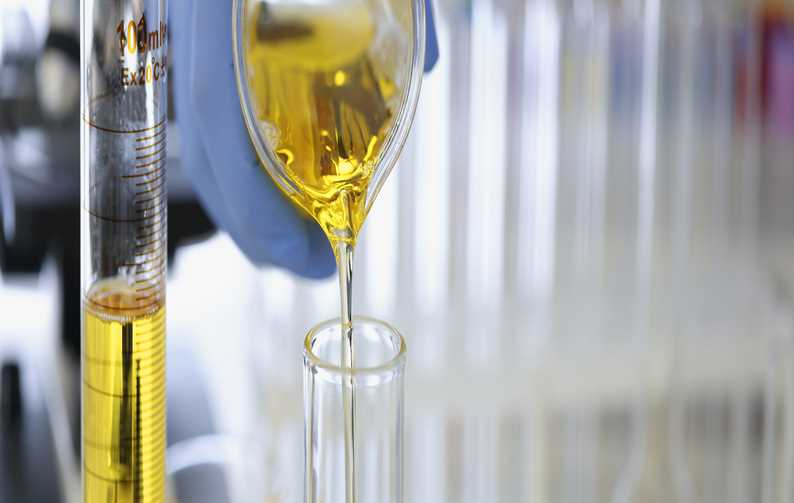# eSource: Test Methodology Sensitivity and Specificity06 JUN 2019 Gary Blevins
Recently I received a number of questions around test methodology on some of the analysis we perform and, in answering these questions, realized that two important properties of testing is not well understood by folks in general, which are Sensitivity and Specificity.

Sensitivity is a measure how responsive the test is to the analyte:

A very sensitive test is one that has a very low Limit of Detection, because it only takes the presence of a very small amount of the analyte to be present in the sample, to obtain a measurable signal against the background noise naturally produced by the test method (good signal to noise ratio).

Specificity is a measure of how many interferences there exist for the test:

A test that has no elements or compounds that can produce a false signal for the analyte has very high specificity, while a test that has several elements or compounds that can produce a false signal for the analyte in question, has very low specificity.

The reason these concepts are poorly understood, is that outside of the medical field, these two properties of a test are not discussed/measured/considered.

So how are these properties of a test determined?

To understand this, let us consider how these two properties relate to each other using this diagram.

1. Our Ideal test has high Sensitivity and high Specificity, this will give the best possible data.

2. A test with high Sensitivity but low Specificity will tend to report false positive results because it responds strongly (readily produces a number from the test) but is easily fooled by what the number represents.

3. A test with low Sensitivity but high Specificity will tend to report false negative results, because the signal is easily confused with background noise.

4. The worst outcome is a test with low Sensitivity and low Specificity which will give us bad data.

Points 2 and 3 give us our 'measure' of these properties of the test. If we can establish the number of 'false positives' a test generates, we can calculate a Sensitivity ratio for the test.

Conversely if we can establish how many 'false negatives' the test is generating then we can calculate a Specificity ratio for the test.

Sensitivity measure is = "Number of measured Positive results" divided by "Total number of Positive results"

This will give us a value between zero and one, for example, if we measure 8 positive results out of 10 samples that should produce a positive result, the Sensitivity of the test is 8/10 = 0.8. The closer the number is to 1, the more sensitive the test is.

Similar with Specificity, the measure is = "Number of measured Negative results" divided by "Total number of Negative results". For example, if we had 12 measured negative results out of a true count of 10 negative results will be 12/10 = 1.2. Again, the movement away from the 'ideal' value "1" tells us how specific a test is to the analyte.

To illustrate these concepts, let us take testing an oil sample for water as an example; there are three common ways to do this test, crackle, FTIR and Karl Fisher. Let's take each by turn and consider the Sensitivity and Specificity of each method.

##### Crackle

Sensitivity; crackle is a high sensitivity test, as little as 0.05% water can be detected if the hotplate is at the optimal temperature.

Specificity; crackle is not specific to water, anything in the oil that boils below the hotplate's temperature can produce a false positive result.

From this knowledge we can predict that the crackle technique is likely to produce 'False positives' for water in our oil samples and a method will be needed to weed them out.

##### FTIR

Sensitivity; FTIR has low sensitivity to water because, as the old saying goes, water and oil doesn't mix so well. This means the water molecules in our oil sample are mostly discrete from the oil molecules, as the laser only energizes a very small portion of the oil, it can miss the water completely.

Specificity; FTIR has high specificity to water, because it can detect the energy absorbed by the oxygen to hydrogen bond and the hydrogen to hydrogen bond, we can tell with a high degree of certainty that we are measuring water.

From this knowledge we can predict that the FTIR technique is likely to produce 'False negatives' for water in our oil samples and we will have to employ other means to detect all samples with water.

##### Karl Fisher

Sensitivity; the Karl Fisher titration is very sensitive to water and can detect down to 0.005% water.

Specificity; Karl Fisher titration is highly specific to water. There are known interferences such as formamide, but as this is unlikely to be in our oil samples.

From this knowledge we can predict that the Karl Fisher technique is unlikely to produce 'False positives' or 'False negatives' for water in our oil samples, making it the ideal method.

Now that you are aware of these two properties of testing, please factor them into the selection of which test methodology is best suited to determine the analytes of interest.

Written By:

Gary Blevins
Technical Director, North America
ALS Tribology